• Post author:

# Natural deduction

Natural deduction System for a structured deduction from a set of assumptions, based on rules, specific to the logical connectives. The way of proving that an argument is valid is to break it down into several steps and to show that everyone can conclude some more obvious and valid arguments. The proof system is defined in purely syntactic terms.

A deduction is a sequence of formulas formed by obeying some fixed rules. For proving theorems in mathematics or any other science, deduction is a simplified model. Deductions are also called formal proofs. If a deduction is correct, it is easy to check. Even a computer can check correctness; the difficulty is just in finding the deduction.

The deduction is a calculus for reasoning about propositions. It is expensive to test whether a proposition is a tautology by testing every possible truth assignment (there are exponentially many). The deductive system will allow us to construct proofs of tautologies in a step-by-step fashion, known as natural deduction. There is a collection of proof rules in natural deduction. That allows us to infer new sentences logically followed from existing sentences or allows us to draw a conclusion given a certain arrangement of premises. These proof rules allow us to infer new formulas from other formulas or allow us to infer a conclusion from a set of premises.

Just suppose there is a set of sentences: φ1, φ2, . . . , φn called premises, and another sentence/formula ψ called a conclusion. By applying proof rules to the premises, it hopes to get some more formulas, and by applying more proof rules to those formulas, it hopes to eventually obtain the conclusion. This intention is denoted by:

φ1, φ2,…,φn |− ψ.

This expression/notation is called a sequent. A sequent is valid if a proof that is built by the proof rules can be found.

In logic, it is traditional to use Greek letters. Lower-case letters are used to stand for formulas and upper-case letters are used to stand for sets of formulas.

#### Natural deduction rules

In natural deduction rules, the propositions above the line are called premises whereas the proposition below the line is the conclusion. Both the premises and the conclusion may contain meta-variables representing arbitrary propositions. The meta-variables are replaced consistently with the appropriate kind of proposition when an inference rule is used as part of a proof. Most of the deduction rules come in one of two flavors, introduction or elimination.  Introduction rules introduce the use of a logical operator whereas and elimination rules eliminate the use of a logical operator. The name of the rule is written on the right-hand side of a rule, which is helpful when reading proofs. There are some introduction rule(s) and elimination rule(s) for each logical connective.
There is also a concept of assumptions such as Introduction (opening) and cancelation (closing, discharge) of assumptions. An Assumption can be re-used many times before its cancelation. An assumption is canceled only when the rules allow it to be but not an obligation however, the less the assumptions, the stronger the derivation.

#### Rules for natural deduction:

##### The rules for conjunction

The first natural deduction rule is called the rule for conjunction (∧): and-introduction. It allows us to conclude φ ∧ ψ, in case we have already concluded φ and ψ separately. This rule is written as: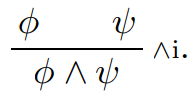Note: premises of the rule are shown above the line and the conclusion is below the line. To the right of the line, ∧i is the name of the proof rule and is read as ‘and-introduction’. Since we have introduced conjunction (∧) in the conclusion where there was none in the premises. There are two rules to eliminate it. These rules are called and-elimination.The rule ∧e1 says: if you have a proof of φ ∧ ψ, then you can get a proof of φ by applying this rule. The rule ∧e2 says: if you have a proof of φ ∧ ψ, then you can get a proof of ψ by applying this rule.

Example: prove that p ∧ q, r |− q ∧ r is valid.Example 2: Prove that the sequent (p ∧ q) ∧ r, s ∧ t |− q ∧ s is valid.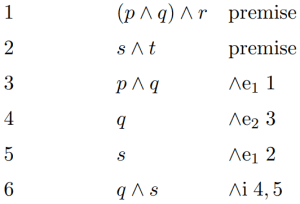##### The rules of double negation

There is no difference, intuitively, between a formula φ and its double negation ¬¬φ.

The sentence: ‘It is not true that today is not Sunday.’ is just a more contrived way of saying.

‘Today is Sunday.’

Conversely, knowing ‘Today is Sunday,’ we can easily state this fact in this more complicated manner if we wish. Thus, the rules of elimination and introduction for double negation are:Example: prove that the sequent p, ¬¬(q ∧ r) |− ¬¬p ∧ r is valid.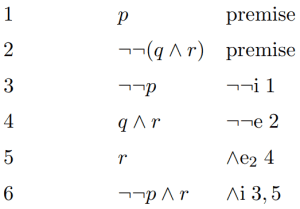##### The rule for eliminating implication

There is one rule to introduce → as well as one to eliminate it. The eliminating implication is one of the best-known rules of propositional logic and is often referred to as “modus ponens” by its Latin name. It is usually called by its modern name, implies-elimination that is sometimes also referred to as arrow-elimination.This rule states that: if φ is given and we knowing that φ implies ψ, then we may rightfully conclude ψ.

Let’s justify this rule by spelling out instances of some declarative sentences φ and ψ. Suppose that

φ: It rained.
φ → ψ: If it rained, then the street is wet.

So ψ is just ‘The street is wet.’, that is the conclusion. Thus, the justification of the ‘→e’ rule is a mere application of common sense.

Example: prove that the sequent p, p → q, p → (q → r) |− r is valid.Here is also a hybrid rule which has the Latin name modus tollens, before turning to implies-introduction, let’s look at this. It eliminates an implication like the, →e rule. Let’s suppose p → q and ¬q are the cases. Then, we can use →e to conclude that q holds, on the behalf of if p holds. Thus, we have that q and ¬q hold, which is impossible. So, we may infer that p must be false. But it means that ¬p is true. This reasoning summarized into the rule modus tollens, or MT for short:Example of this rule in the natural language setting:

‘If Abraham Lincoln was Ethiopian, then he was African. Abraham Lincoln was not African; therefore he was not Ethiopian.’

Example: prove that the sequent p → (q → r), p, ¬r |− ¬q is valid.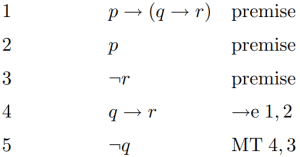Example: prove that the sequent ¬p → q, ¬q |− p is valid.Example: prove that the sequent p → ¬q, q |− ¬p is valid.##### The rule implies the introduction

Suppose that p → q is the case. By temporarily assuming that ¬q holds, MT rule can be used to infer ¬p. Thus, by assuming p → q we can show that ¬q implies ¬p, symbolically as ¬q → ¬p. To summarize here is an argumentation for p → q |− ¬q → ¬p:The box in the proof serves to demarcate the scope of the temporary assumption ¬q. That we usually say: let’s assume ¬q. For this, we open a box and put ¬q at the top. Then we continue by applying other rules as normal, e.g., to obtain ¬p. But this entire still depends on the assumption of ¬q, so it goes inside the box. And finally, we are ready to apply →i. That allows us to conclude ¬q → ¬p, but the conclusion (¬q → ¬p) no longer depends on the assumption ¬q.

Thus we formulate the rule →i, as follows:This rule says: to prove φ → ψ, first make a temporary assumption of φ and then prove ψ. In the proof of ψ, you can use φ and any of the other formulas such as premises and provisional conclusions that you have made so far. Proofs may nest the boxes or open new boxes after old boxes that have been closed.

Example: prove that the sequent ¬q → ¬p |− p → ¬¬q is valid.Example: prove that the sequent |− p → p is valid.This proof is to express that the argumentation for p → p does not depend on any premises at all.

Example: prove that the sequent p ∧ q → r |− p → (q → r) is valid.Example:  prove that the sequent p → (q → r) |− p ∧ q → r is valid.This shows that the ‘converse’ of the sequent above is valid, too.
The validity of p → (q → r) |− p ∧ q → r and p ∧ q → r |− p → (q → r) in above two proofs shows that these two formulas are equivalent in the sense that we can prove one formula from the other formula and denote as:

p ∧ q → r p → (q → r).

There can be only one formula to the right of, whereas each instance can only relate two formulas to each other.

Example: prove that the sequent p → q |− p ∧ r → q ∧ r is valid.##### The rules for disjunction

The rules for disjunction are different from those for conjunction, in spirit. For conjunction the case was concise and clear. The proof of φ ∧ ψ is essentially nothing; it’s just a concatenation of a proof of φ and a proof of ψ plus an addition of a line invoking ∧i. However, in the case of disjunction, it turns out that elimination is a tremendous task. We can infer that ‘φ or ψ’ holds, from the only premise φ, as we already know that φ holds. The point to be noticed here is that this inference is valid for any choice of ψ. We may conclude ‘φ or ψ’ by the same token if we already have ψ. And similarly, that inference also works for any choice of φ. Thus we found the proof rules ∨i1 and ∨i2.Let’s consider or-elimination now. Let’s imagine that we want to show some proposition χ by assuming φ ∨ ψ. Since we exactly don’t know which of φ and ψ is true, so we must have to give two separate proofs which we need to combine into one argument:

• At first, we assume φ is true and have to come up with a proof of χ.
• And next, we assume ψ is true and need to give a proof of χ as well.
• Then we can infer χ from the truth of φ ∨ ψ.

So, we write the rule ∨e as follows:It is saying that: if φ ∨ ψ is true (and no matter whether we assume φ or we assume ψ), we can get a proof of χ

Example: prove that the sequent p ∨ q |− q ∨ p is valid.• make sure that the conclusions are the same formula in each of the two cases
• The work done by the or-elimination rule ∨e is the combining of the arguments of the two cases into one.
• In each case, you may use the temporary assumption of the other case, only if it is something that has already been shown before those case boxes began (not as an assumption).
• The invocation of rule ∨e-lists three things: the location of the two boxes for the two cases and the line in which the disjunction appears.

Example: prove that the sequent q → r |− p ∨ q → p ∨ r is valid.Example: prove that the sequent (p ∨ q) ∨ r |− p ∨ (q ∨ r) is valid.Example: prove that the sequent p ∧ (q ∨ r) |− (p ∧ q) ∨ (p ∧ r) is valid.##### The rules for negation

The particular fact that ⊥ can prove anything is encoded in our calculus by the proof rule bottom-elimination, given as:The other fact that ⊥ itself represents a contradiction is encoded in our calculus by the proof rule not-elimination, given as: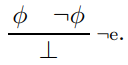Example: prove that the sequent ¬p ∨ q |− p → q is valid (using the above two rules).The proof boxes for ∨e are drawn side by side to show that, doesn’t matter which way you do it.

Now for introducing negations, suppose we make an assumption which gets us into a contradictory state of affairs, i.e. ⊥. In that case, our assumption cannot be true; so it must be false. This intuition is the basis for the negation introduction proof rule written as ¬i:Example: prove that the sequent p → q, p → ¬q |− ¬p is valid.Example: prove that the sequent p → ¬p |− ¬p is valid.Example: prove that the sequent p → (q → r), p, ¬r |− ¬q is valid (without using the MT rule).Example: prove that the sequent p ∧ ¬q → r, ¬r, p |− q is valid.##### Derived rules

Here are some rules that are extremely useful and are not primitive rules of natural deduction, but can be derived from some of the other rules. Modus tollens is one of these rules.Here is the derivation of the proof rule Modus tollens (MT) from →e, ¬e, and ¬i:We can replace applications of MT by this combination of →e, ¬e, and ¬i, in the above proofs.

The same holds for the rule double negation introduction:That can be derived from the rules ¬i and ¬e, as follows:The next derived rule has the Latin name “reductio ad absurdum” which means ‘reduction to absurdity’ and we may simply call it proof by contradiction (PBC for short).The rule says: if we obtain a contradiction from ¬φ, then we are entitled to deduce φ:

Suppose that we have a proof of ⊥ from ¬φ and by →i, we can transform this into a proof of ¬φ → ⊥. So the following derivation shows that PBC can be derived from →i, ¬i, →e, and ¬¬e.The final derived rule we consider in this section has a Latin name, “tertium non datur”; the English name is the law of the excluded middle or LEM for short. It is arguably the most useful to use in proofs, because its derivation is rather long and complicated, so its usage most often saves time and effort.

It says that φ ∨ ¬φ is true: whatever φ is, it must be either true or false; in the latter case, ¬φ is true. There is no third possibility (hence excluded middle): the sequent |− φ ∨ ¬φ is valid.

Here is the natural deduction proof that derives the law of the excluded middle from basic proof rules: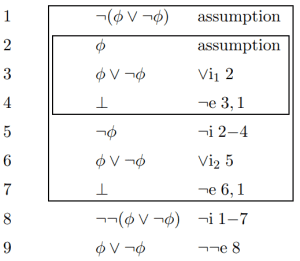Example: Using LEM proves that the sequent p → q |− ¬p ∨ q is valid.#### Provable equivalence

Let φ and ψ be formulas of the propositional logic. φ and ψ are provably equivalent iff (if, and only if) the sequents φ |− ψ and ψ |− φ are valid, that is, there is a proof of ψ from φ and also a proof of φ from ψ. It is called as φ and ψ are provably equivalent and denoted as φ−||− ψ.

Some examples of provably equivalent formulas are:Propositional logic

### This Post Has 2 Comments

1.Apex Legends Season 1

I like reading an article that can make men and women think. Also, thank you for permitting me to comment!

2.zvodretiluret

We’re a group of volunteers and opening a new scheme in our community. Your web site offered us with valuable information to work on. You’ve done a formidable job and our entire community will be grateful to you.